### Problem Statement

You need to write a program to race a car. The car will drive along a track, as defined by a sequence of waypoints (you don't actually have to stay on the track, you just have to hit the waypoints).

You will first be given a number of parameters relating to the performance of the car, in a method init, along with the locations of the waypoints. Your car starts out at (0,0), facing in the +x direction. A number of calls will then be made to a method step, reminding you of your current location and direction. You should return a double[] from this method, where consecutive elements of the return indicate first how much to accelerate or break, and then how much to turn the car (in radians), with the acceleration element coming first. Each turning element must have absolute value no more than 0.005, and each accelerating element must have absolute value no more than maxacc. The car's motion will be simulated in a number of discrete timesteps, corresponding to your return. Thus, a return with 6 elements, would result in the simulation running for 3 steps.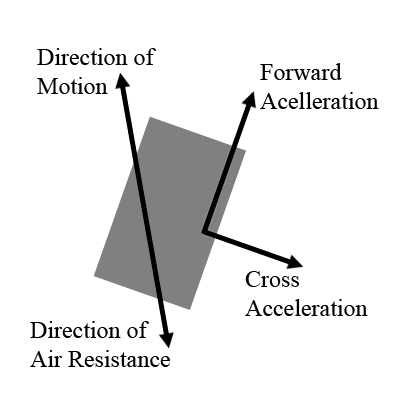The physics of our car's motion are fairly simple (and perhaps not too realistic). The current state of the car is (mostly) defined by five values: it's x and y positions, it's x and y velocities, and the angle it is facing. It should be noted that the car may be facing in a different direction from the one it is moving in, as it might skid around a turn, or even roll backwards.

In a particular time step, you apply a forward force of up to maxacc to the car, propeling it in the direction it is facing. Air resistance works to counter that force, and the net forward force is given by the force you apply minus the forward air resistance (which is proportional to velocity squared). If the car is not moving in the same direction it is facing, there is also a cross force, which can be as large as the car's velocity in this cross direction. Finally, you can apply the breaks, slowing the car down up to maxacc in the forward direction, by returning a negative value for acceleration.

The friction of the tires can only provide a limited amount of force, which must be distributed between the cross force and the forward force. If the magnitude of the overall force vector is larger than the force of friction, then the car is said to be skidding. In this case, the force of friction is divided between the forward and cross directions. At the end of each time step, after all the forces have been computed, the car's velocity is first adjusted, and then the car's position is adjusted according to this new velocity. Finally, if the car was skidding in the previous timestep, it builds some angular momentum, and the change in it's angle will be set to whatever change you impart, plus half of the change from the previous timestep.

All this adds up to fairly intuitive motion. If you don't turn too sharply and you don't accelerate too much, the force of friction is large enough to entirely adjust the cars velocity so that it is moving in the direction it is facing, and no skidding occurs. If you turn too sharply, there isn't enough friction on the tires, and you will start to skid. In this case, the car will still turn, but it won't be moving in the direction it is facing. In extreme cases, you could turn the car all the way around, and end up rolling backwards. The amount of acceleration you can apply without exceeding the force of friction increases with your speed (so its more like power than acceleration), as air resistance is removed from forward acceleration before checking if there is enough friction.

To complete the race, you must come within 1000 units of each of the waypoints, in order. The simulation will be run for at most 100,000 steps and you will be allowed a total of 30 seconds for each test case.

### Test Case Generation

The friction parameter will be uniformly chosen in [0.002,0.03]. The air resistance will be chosen in [0.0001,0.001], and the maximum acceleration will be chosen in [0.01,0.1]. The waypoints will be generated by starting at (0,0), facing in the +x direction. A sequence of moves and turns will then be made, placing a waypoint after each:
```        S = nextInt(91)+10;
double alpha = 0;
double cx = 0, cy = 0;
wx = wy = 0;
for(int i = 1; i<S; i++){
alpha += nextGaussian()/2;
double d = pow(nextDouble(),2) * 5000;
wx[i] = cx += Math.cos(alpha)*d;
wy[i] = cy += Math.sin(alpha)*d;
}
```

### Scoring

Your raw score will be the timestep at which you hit the last of all the waypoints that you hit, plus the number of waypoints hit divided by 1000. Competitors will be ranked primarily by the number of waypoints hit (you should be able to always hit all of them), with ties broken by the speed with which you hit them. The highest rank is 1, and each competitor will get (12-rank)2 points. Thus, the winner will get 121 points. In the event of a tie, the points for the tied places will be divided evenly. Thus, if there were a tie for first, the top two would each get (121+100)/2 points, while third would still get 81.

### Resources

You can see the source code and a more detailed explanation of the physics at http://www.topcoder.com/contest/problem/CarRace/physics.html.

There is also a visualizer, described at http://www.topcoder.com/contest/problem/CarRace/vis.html.

### Definition

 Class: CarRace Method: init Parameters: double, double, double, double[], double[] Returns: int Method signature: int init(double friction, double air, double maxacc, double[] wx, double[] wy) Method: step Parameters: double, double, double, double, double, int Returns: double[] Method signature: double[] step(double x, double y, double dx, double dy, double alpha, int wp) (be sure your methods are public)

### Notes

-Breaking while going backwards still makes you stop -- it doesn't accelerate you in reverse.
-The time limit is 30 seconds and the memory limit is 512M.
-You will have at most 100,000 steps to finish. If you don't finish all waypoints, your score will be as described in the problem, and you will be ranked behind everyone who hit more waypoints than you.
-The direction you are facing will be between -PI and PI. You will start facing in the +x direction, with alpha = 0. Turning clockwise corresponds to increasing alpha.

### Constraints

-In each step you may turn any amount between -0.005 and 0.005.
-In each step you may accelerate an amount between -maxacc and maxacc

### Examples

0)

 `"1"`
```Returns:
"friction = 0.025353231925443906<br>
air = 4.965840810810231E-4<br>
maxacc = 0.010020588605228068<br>
N = 85<br>
"```1)

 `"2"`
```Returns:
"friction = 0.026415714551864464<br>
air = 1.4666721060721836E-4<br>
maxacc = 0.01893924570669956<br>
N = 92<br>
"```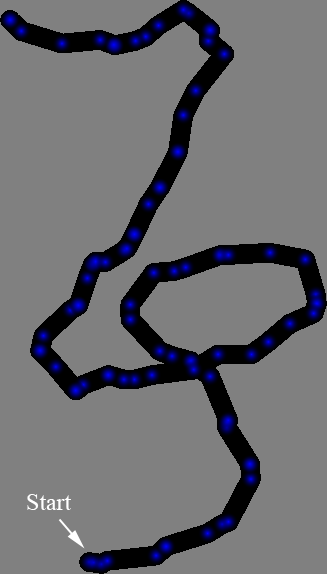2)

 `"3"`
```Returns:
"friction = 0.004844682646896966<br>
air = 4.746660802103152E-4<br>
maxacc = 0.06236285385761371<br>
N = 10<br>
"```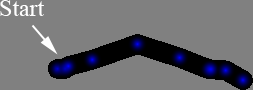3)

 `"4"`
```Returns:
"friction = 0.02815367095844578<br>
air = 1.8501805598421562E-4<br>
maxacc = 0.09508318371791942<br>
N = 97<br>
"```4)

 `"5"`
```Returns:
"friction = 0.014431617598556953<br>
air = 7.673181465344457E-4<br>
maxacc = 0.04720944775523275<br>
N = 26<br>
"```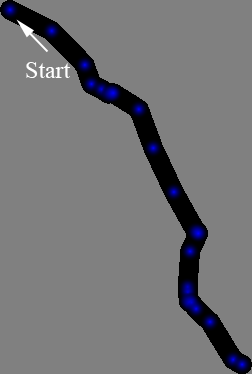5)

 `"6"`
```Returns:
"friction = 0.02400016822715227<br>
air = 6.975636481665687E-4<br>
maxacc = 0.06782124248871851<br>
N = 89<br>
"```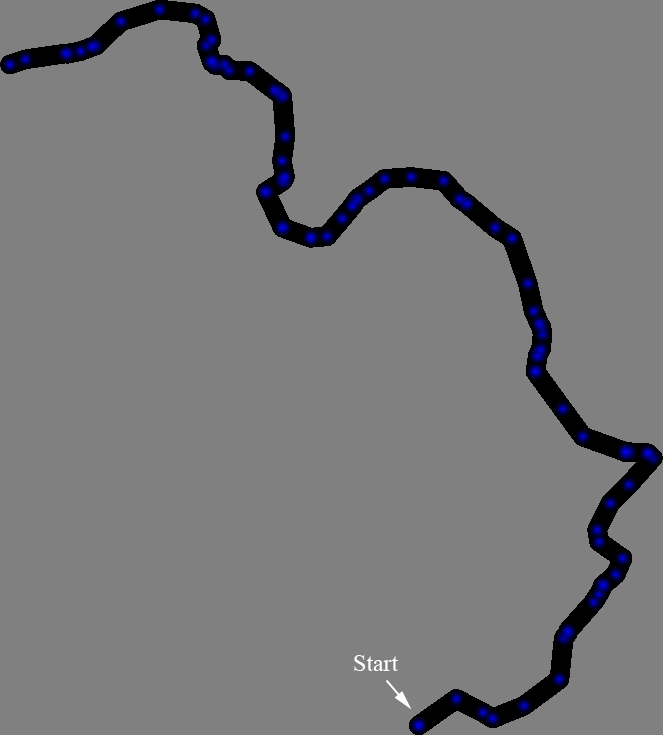6)

 `"7"`
```Returns:
"friction = 0.0062732643115944176<br>
air = 6.03853832499194E-4<br>
maxacc = 0.08891366051327443<br>
N = 33<br>
"```7)

 `"8"`
```Returns:
"friction = 0.022912046591109785<br>
air = 9.433731971918565E-4<br>
maxacc = 0.07645501697340658<br>
N = 18<br>
"```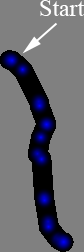8)

 `"9"`
```Returns:
"friction = 0.0025809528661937535<br>
air = 1.0337427216509109E-4<br>
maxacc = 0.09923919367667183<br>
N = 32<br>
"```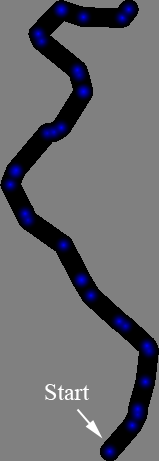9)

 `"10"`
```Returns:
"friction = 0.017193956023422358<br>
air = 1.3735350430489604E-4<br>
maxacc = 0.034056681729733335<br>
N = 38<br>
"```10)

 `"11"`
```Returns:
"friction = 0.012095102876343383<br>
air = 1.350554326067197E-4<br>
maxacc = 0.09337933523810507<br>
N = 23<br>
"```11)

 `"12"`
```Returns:
"friction = 0.01063311914214325<br>
air = 5.320894448422347E-4<br>
maxacc = 0.057396703337113456<br>
N = 13<br>
"```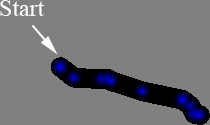12)

 `"13"`
```Returns:
"friction = 0.017551334976223812<br>
air = 5.275741890291596E-4<br>
maxacc = 0.06837013496585978<br>
N = 35<br>
"```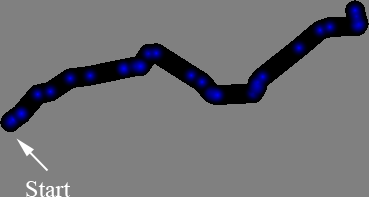13)

 `"14"`
```Returns:
"friction = 0.002780827021052145<br>
air = 5.916970900662024E-4<br>
maxacc = 0.07667698326331136<br>
N = 38<br>
"```14)

 `"15"`
```Returns:
"friction = 0.02153379082007357<br>
air = 4.2201266203282357E-4<br>
maxacc = 0.019785379399535032<br>
N = 38<br>
"```15)

 `"16"`
```Returns:
"friction = 0.014504300422171476<br>
air = 1.4169402429845034E-4<br>
maxacc = 0.019126261838502728<br>
N = 78<br>
"```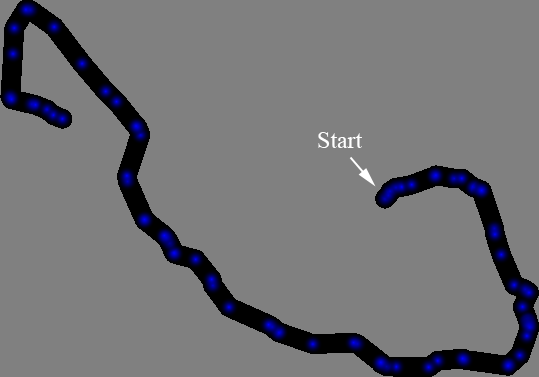16)

 `"17"`
```Returns:
"friction = 0.018501240274952514<br>
air = 1.5505615534243216E-4<br>
maxacc = 0.04447374141309209<br>
N = 55<br>
"```17)

 `"18"`
```Returns:
"friction = 0.010420957638070267<br>
air = 1.098160773416636E-4<br>
maxacc = 0.07814826571569722<br>
N = 56<br>
"```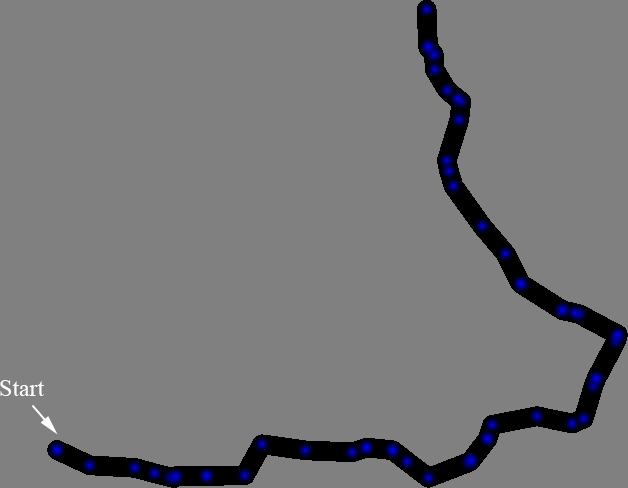18)

 `"19"`
```Returns:
"friction = 0.007461881907706251<br>
air = 5.702494936263828E-4<br>
maxacc = 0.05444883456395739<br>
N = 62<br>
"```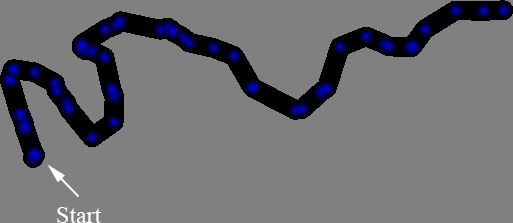19)

 `"20"`
```Returns:
"friction = 0.006935324539288438<br>
air = 8.158847041045977E-4<br>
maxacc = 0.08047553123971915<br>
N = 19<br>
"```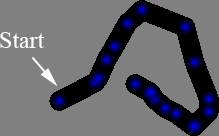#### Problem url:

http://www.topcoder.com/stat?c=problem_statement&pm=8816

#### Problem stats url:

http://www.topcoder.com/tc?module=ProblemDetail&rd=12020&pm=8816

Unknown

Simulation